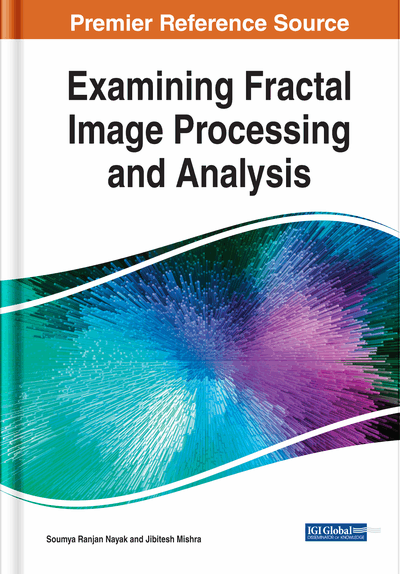# Empirical Performance Analysis of Wavelet Transform Coding-Based Image Compression Techniques

Tawheed Jan Shah (University of Kashmir, India) and M. Tariq Banday (University of Kashmir, India)
DOI: 10.4018/978-1-7998-0066-8.ch004
Available
\$37.50
No Current Special Offers

## Abstract

In this chapter, the performance of wavelet transform-based EZW coding and SPIHT coding technique have been evaluated and compared in terms of CR, PSNR, and MSE by applying them to similar color images in two standard resolutions. The application of these techniques on entire color images such as passport size photograph in which the region containing the face of a person is more significant than other regions results in equal loss of information content and less compression ratio. So, to achieve the high CRs and distribute the quality of the image unevenly, this chapter proposes the ROI coding technique. Compressing ROI portion using discrete wavelet transform with Huffman coding and NROI compressed with Huffman, EZW coding, SPIHT coding suggested effective compression at nearly no loss of quality in the ROI portion of the photograph. Further, higher CR and PSNR with lower MSE have been found in high-resolution photographs, thereby permitting the reduction of storage space, faster transmission on low bandwidth channels, and faster processing.
Chapter Preview
Top

## Introduction

### Wavelet-Based Image Compression

Wavelets were introduced as a signal processing tool in the late 1980s. Since then considerable attention has been given on the application of wavelets to Image compression (Woods & ONeil, 1986). Wavelet image coding follows a well-understood standard transform coding prototype (Lewis & Knowles, 1992). Foremost, the wavelet transform is applied on an image then the wavelet coefficients are quantized and later coded by applying lossless coding on the quantized coefficients. However, this transform gives a multi-resolution and multi-scale representation of the image. The wavelet transform uses wavelets of fixed energy to examine the transient, time-variant signals. A Wavelet is thus defined as an irregular mathematical function having limited duration effectively. The word ‘wavelet’ is used because it integrates to zero (Zhao et al., 2004).

One of the most crucial advantages of the wavelet is its ability to analyze the localized area of a large signal (Schelkens et al., 1999). Small wavelet can be used to isolate the fine details of the signal, and the large wavelet can identify the coarse details. Assume the sine wave generated in the real world by a noisy switch or power fluctuation with a small discontinuity which is hardly noticeable as shown in Figure 1. However, with the help of the wavelet coefficient plot, the exact location of the discontinuity can be found.

The wavelet transform (WT) of a signal is its time-frequency representation, and this transform does not change the information content of the signal. It is computed independently for different segments of the signal in time-domain at different frequencies. It gives good frequency resolution and poor time resolution at lower frequencies while at higher frequencies, it gives good time resolution and poor frequency resolution. The WT was developed to overcome the shortcomings of STFT (Sifuzzaman et al., 2009). The basis sine and wavelet waveform are shown in Figure 2.

It can be seen intuitively that wavelet waveform might analyze the sharply changing signal better than a sine waveform, just as a fork handles some foods better than a spoon.

There are two types of Wavelet Transforms namely, Continous wavelet transform (CWT) and discrete wavelet transform and are explained below:

## Key Terms in this Chapter

CWT: Continous Wavelet Transform

EZW: Embedded Zero Tree Wavelet

STFT: Short Time Fourier transform

HPF: High Pass Filter

HVS: Human Visual System

JPEG: Joint Photographic Experts Group

BPP: Bits Per Pixel

NROI: Non-Region of Interest

MSE: Mean Square Error

WT: Wavelet Transform

SPIHT: Set Partitioning in Hierarchical Trees

PSNR: Peak Signal to Noise Ratio

LPF: Low Pass Filter

ROI: Region of Interest

## Complete Chapter List

Search this Book:
Reset